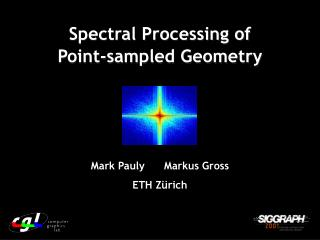DownloadDownload PresentationSpectral Processing of Point-sampled Geometry

# Spectral Processing of Point-sampled Geometry

Télécharger la présentation## Spectral Processing of Point-sampled Geometry

- - - - - - - - - - - - - - - - - - - - - - - - - - - E N D - - - - - - - - - - - - - - - - - - - - - - - - - - -
##### Presentation Transcript

1. Spectral Processing of Point-sampled Geometry Mark Pauly Markus Gross ETH Zürich

2. Outline • Introduction • Spectral processing pipeline • Results • Conclusions

3. Introduction Point-based Geometry Processing Spectral Methods Introduction • Model • Acquisition • Range scans • Depth images • … • Point • Rendering • QSplat • Surfels • …

4. Introduction Spectral Transform • Extend Fourier transform to 2-manifold surfaces • Spectral representation of point-based objects • Powerful methods for digital geometry processing

5. Introduction Applications • Spectral filtering: • Noise removal • Microstructure analysis • Enhancement • Adaptive resampling: • Complexity reduction • Continuous LOD

6. Introduction Fourier Transform • Benefits: • Sound concept of frequency • Extensive theory • Fast algorithms • Limitations: • Euclidean domain, global parameterization • Regular sampling • Lack of local control

7. Spectral Processing Pipeline Overview

8. Spectral Processing Pipeline Patch Layout Generation Clustering  Optimization Samples  Clusters  Patches

9. Spectral Processing Pipeline Patch Merging Optimization • Iterative, local optimization method • Quality metric: patch Size  curvature  patch boundary  spring energy regularization

10. Spectral Processing Pipeline Hierarchical Push-Pull Filter: Scattered Data Approximation

11. Spectral Processing Pipeline Spectral Analysis • 2D Discrete Fourier Transform (DFT) • Direct manipulation of spectral coefficients • Filtering as convolution: • Convolution: O(N2)  Multiplication: O(N) • Inverse Fourier Transform • Filtered patch surface

12. Spectral Processing Pipeline Spectral Analysis Original Gaussian low-pass Ideal low-pass Band-stop Enhancement

13. Spectral Processing Pipeline Resampling • Low-pass filtering • Band-limitation • Regular Resampling • Optimal sampling rate (Sampling Theorem) • Error control (Parseval’s Theorem) Power Spectrum

14. Spectral Processing Pipeline Reconstruction • Filtering can lead to discontinuities at patch boundaries • Create patch overlap, blend adjacent patches region of overlap Sampling rates Point positions Normals

15. Spectral Processing Pipeline

16. Results Surface Restoration Original Gaussian Wiener Patch noise+blur Filter Filter Layout

17. Results Interactive Filtering

18. Results Adaptive Subsampling 4,128,614 pts. = 100% 287,163 pts. = 6.9%

19. Results 9% 38% 23% 4% 26% Timings Clustering Time Patch Merging SDA Analysis Reconstruction

20. Results Timings

21. Conclusions Summary • Versatile spectral decomposition of point-based models • Effective filtering • Adaptive resampling • Efficient processing of large point-sampled models

22. Conclusions Future Work • Compression • Scalar Representation + Spectral Compression • Hierarchical Representation • Modeling and Animation • Feature Detection & Extraction • Robust Computation of Laplacian

23. Acknowledgements Our Thanks to: Marc Levoy and the Stanford Digital Michelangelo Project, Szymon Rusinkiewicz, Bernd Gärtner